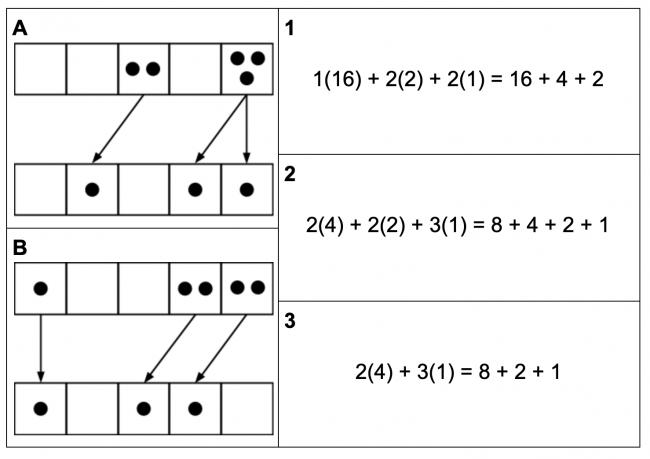# Exploding Dots

Preview:This task develops relationships between arithematic and the Exploding Dots representation by James Tanton.

Connecting Representations
Big Idea:
Grade 9: Computational fluency and flexibility with numbers extend to operations with rational numbers.
Content:
exponents and exponent laws with whole-number exponents
Curricular Competency:
Reasoning and analyzing: Use reasoning to explore and make connections
Reasoning and analyzing: Develop mental math strategies and abilities to make sense of quantities
Understanding and solving: Develop, demonstrate, and apply mathematical understanding through play, inquiry, and problem solving
Understanding and solving: Visualize to explore mathematical concepts
Communicating and representing: Communicate mathematical thinking in many ways